main | forumNovember 28th, 2023CIS 751MainSyllabusOverviewForumUPLOAD PROJECTSNotes00010002000300040005000600070008000900100011001200130014SIGNAL NotesDatabase NotesFinal(Take Home)# Introduction

First of all, this is not a math course, and we will not spend much time dealing with mathematical details. The course is based on intuition, not mathematical details. That being said, here are some math review material that you might find useful in conceptualizing some of the material in this class.

## Pi, Sin, Cos

Besides for basic operations like addition/subtraction, multiplication/division, we also need to worry about trigonometry. These mostly revolve around the value of Pi, and functions Sin and Cos.

The value of Pi (first ~1000 digits) is:

3.1415926535897932384626433832795028841971693993751058209749445923078164062862 089986280348253421170679821480865132823066470938446095505822317253594081284811 174502841027019385211055596446229489549303819644288109756659334461284756482337 867831652712019091456485669234603486104543266482133936072602491412737245870066 063155881748815209209628292540917153643678925903600113305305488204665213841469 519415116094330572703657595919530921861173819326117931051185480744623799627495 673518857527248912279381830119491298336733624406566430860213949463952247371907 021798609437027705392171762931767523846748184676694051320005681271452635608277 857713427577896091736371787214684409012249534301465495853710507922796892589235 420199561121290219608640344181598136297747713099605187072113499999983729780499 510597317328160963185950244594553469083026425223082533446850352619311881710100 031378387528865875332083814206171776691473035982534904287554687311595628638823 537875937519577818577805321712268066130019278766111959092164201989380952572010

Pi is an irrational number (meaning that you can't write it down exactly, as a fraction). The best you can do is approximate it.

The value of Pi is actually how many diameters it takes to go around a circle.

A useful graphic to remember is the unit circle: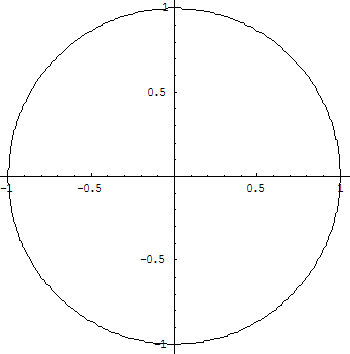You can imagine the X axis being the Cos, and Y axis being the Sin. (ie: Cos(0) = 1, and Sin(0) = 0). Here's the Sin function: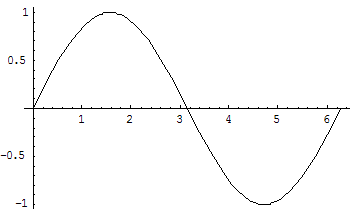And here's the Cos function: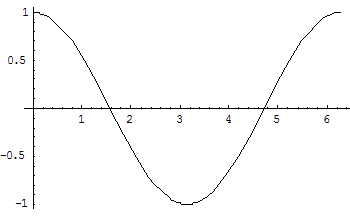(notice that they're mostly the same, except for the phase shift).

## Imaginary Numbers

Imaginary numbers aren't really imaginary. Or to put it another way, all numbers are imaginary, so we might as well not think of imaginary numbers as anything magical. An imaginary number is just sqrt(-1). That's it. Often (to be confusing?) people write it as i.

So for example, if you have something like sqrt(-25), then you can write it as sqrt(25)*sqrt(-1), which nicely translates into 5*sqrt(-1) or 5i.

Well, obviously you can't compute sqrt(-1), but you can still use these things in your calculations, and once you start working with them, interesting things start to happen. For example, what's i squared? ie: sqrt(-1)*sqrt(-1) is... -1.

so...
i0 = 1
i1 = i
i2 = -1
i3 = -i
i4 = 1
i5 = i
i6 = -1
i7 = -i
i8 = 1
i9 = i
i10 = -1
i11 = -i
i12 = 1
...

Notice that the pattern continues to repeat. You can visualize it as a circle: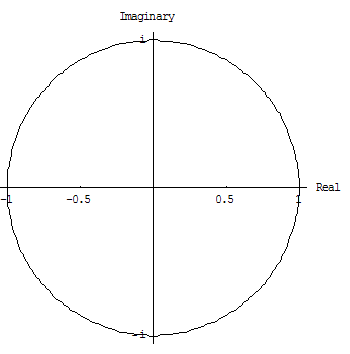## Complex Numbers

A complex number is just a `real' number plus an imaginary number. It is often seen as a tuple, with a real part and an imaginary part. ie: (a + bi), where a is a real part, and bi is the imaginary part. Notation for complex numbers often avoids the + sign; just write: (a, bi).

There really isn't anything `complex' about complex numbers (just like there isn't anything `imaginary' about imaginary numbers). Both are just often convenient number systems.

How would you define addition/subtraction of complex numbers? (btw: It is best not to try to memorize this.)

Lets add (a+bi) and (c+di). What would be the result? For `easier' handling, we can `expand' that into: (a+b*sqrt(-1)) and (c+d*sqrt(-1)).

Drop the parenthesis: a + b*sqrt(-1) + c + d*sqrt(-1), and you end up with: a+c + (b+d)*sqrt(-1), or simply a+c + (b+d)i.

So, to put it simply, addition is defined as: (a, bi) + (c, di) = (a+c, (b+d) i). For example, to add (5, 7i) and (8, 3i), you'd get: (5+8, (7+3)i), or simply (13, 10i). Easy, isn't it? Now come up with subtraction...

Multiplication is a bit more involved, but follows the same idea (again, don't memorize the equation; memorize how we get it): Lets say we want to multiply (a+bi) and (c+di). Let us `expand' it for clarity:  (a+b*sqrt(-1)) and (c+d*sqrt(-1)).

So what we want to do is: (a + b*sqrt(-1)) * (c + d*sqrt(-1)).

Now you just multiply it out:
(a * c + a * d * sqrt(-1) + c * b * sqrt(-1) + b * d * sqrt(-1) * sqrt(-1)).
We know that sqrt(-1) * sqrt(-1) is just -1, so we can replace that part with it:
(a * c + a * d * sqrt(-1) + c * b * sqrt(-1) + b * d * -1),
or, moving things around:
((a * c - b * d) + (a * d + c * b) * sqrt(-1)),
which is just:
((a * c - b * d) + (a * d + c * b)i),
which is the definition for multiplication.

Division is even more involved, and won't be described here.

Now, something really interesting starts to happen when we move to polar coordinate system; what we end up with is this: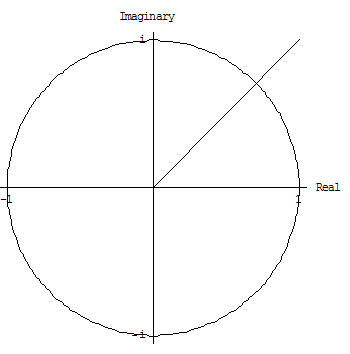You can view the number (a, bi) as a location x,y, where x=a and y=b.

The angle of the `line' to that point is calculated by arctan(a/b), or tan-1(a/b), whichever notation you prefer. The radius of the line is just the distance to that point, which is sqrt(a2 + b2)

Going back is easy too... a = radius * cos(angle), and b = radius * sin(angle).

And now for the interesting bit: If you do multiplication in polar coordinates, it turns out into addition of angles, and multiplication of radiuses. ie:
(r1, theta1) * (r2, theta2) = (r1*r2, theta1+theta2)

Now, consider that the radius is always 1 (like above picture). We can now easily find what are called nth root of unity. For example, slice up the circle into n equal sized chunks. Lets say n=8, so each chunk is 45 degrees. Now, if you multiply out all those 8 distinct `numbers' (on the circle) then you'll end up with (1, 0) - since all the angles will add up to 2Pi, which is equivalent to angle 0.

Roots of unity are useful when calculating Fourier Transforms.